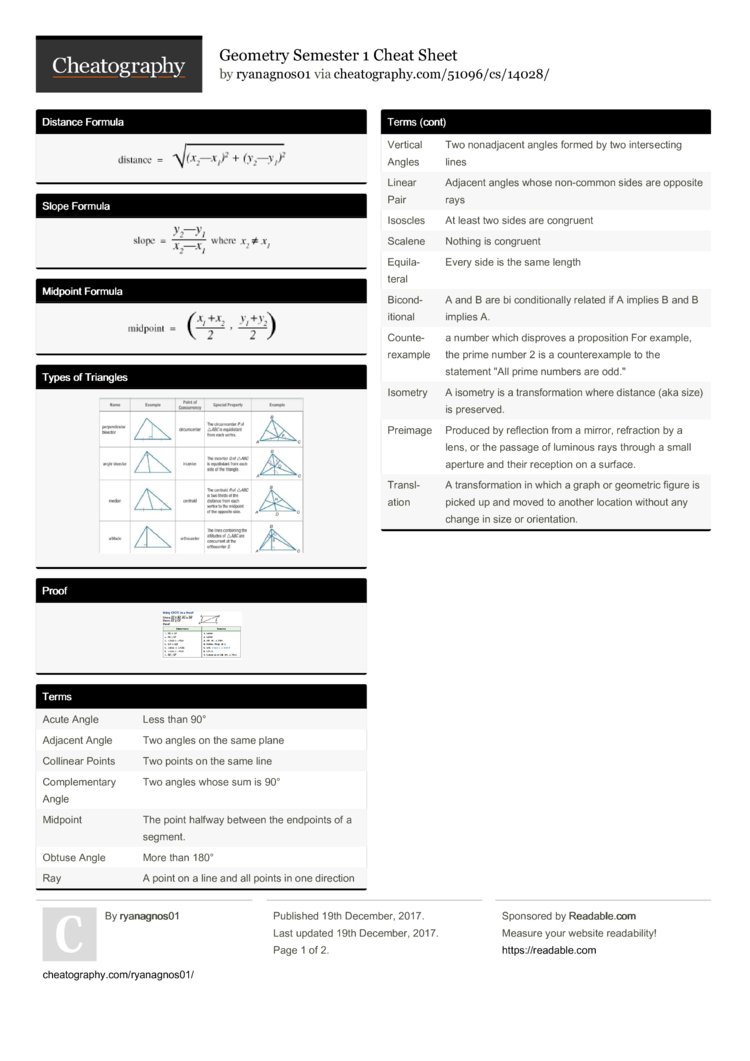# Geometry Semester 1 Cheat Sheet by ryanagnos01

### Distance Formula### Slope Formula### Midpoint Formula### Types of Triangles### Proof### Terms

 Acute Angle Less than 90° Adjacent Angle Two angles on the same plane Collinear Points Two points on the same line Comple­mentary Angle Two angles whose sum is 90° Midpoint The point halfway between the endpoints of a segment. Obtuse Angle More than 180° Ray A point on a line and all points in one direction Vertical Angles Two nonadj­acent angles formed by two inters­ecting lines Linear Pair Adjacent angles whose non-common sides are opposite rays Isoscles At least two sides are congruent Scalene Nothing is congruent Equila­teral Every side is the same length Bicond­itional A and B are bi condit­ionally related if A implies B and B implies A. Counte­rex­ample a number which disproves a propos­ition For example, the prime number 2 is a counte­rex­ample to the statement "All prime numbers are odd." Isometry A isometry is a transf­orm­ation where distance (aka size) is preserved. Preimage Produced by reflection from a mirror, refraction by a lens, or the passage of luminous rays through a small aperture and their reception on a surface. Transl­ation A transf­orm­ation in which a graph or geometric figure is picked up and moved to another location without any change in size or orient­ation.

### Types of Angles### Pythag­orean Theorem### Congruent Angles### Reflec­tions### Proof2 Pages
//media.cheatography.com/storage/thumb/ryanagnos01_geometry-semester-1.750.jpg

PDF (recommended)